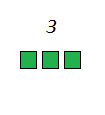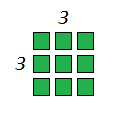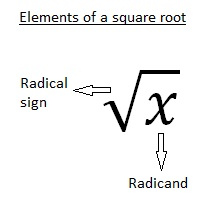# Squares and square roots

Squares and square roots are basic mathematical terms that you will encounter very often, especially in functions and different equations. They are easy to understand and once you figure them out, a new door into the world of exponents and more complex mathematics will open for you.

## Squares

Squares or perfect squares (as they are sometimes called) are the result of numbers multiplied with themselves. So for example, the square of number 3 would be number 9 because 3 times 3 is 9. For the same reason, the square of number 4 would be 16, for number 5 it would be 25 and so on. We will illustrate this to make it a bit clearer.Here we have 3 small squares. If we take two more rows like that, we can arrange them in a perfect square. That big square consists of 9 small squares. In fact, a number is considered a square number only if you can arrange that number of points in a square. Like this:There are a couple of important things to remember. The square root of 1 is 1 (no surprise there because 1*1=1). A square always has a positive value (because a negative number multiplied with a negative number always gives a positive result). The usual notation for the square of a number (represented here with the variable x) is x2.

Also, a handy bit of information is that the square of an even number is also an even number (generic cialis online). It is also divisible by four. No exceptions. And the square of an odd number is always an odd number.

## Square roots

Square roots are the inverse functions of squares. A square root of a number is the number you multiplied with itself to get the number whose square you are looking for. Sounds complicated? Let me rephrase that. If we had a square with an area of A, the square root of A would be the length of one side of that square. That means that, the square root of number 36 would be 6. But also, the square root of number 36 is (-6). It is important to remember that every positive real number has two square roots – one positive and one negative.

Since there are no negative square numbers, real square roots of negative numbers do not exist. In fact, the square root of -1 (and all other negative numbers) is called an imaginary number (i). But we will get to them a bit later, after we post the lessons necessary for understanding them. For now – take our word for it.

The unique, non negative square root of every real number is called the principal square root. The term whose root we are interested in is called the radicand.These are the basic things about squares and square roots. If you wish to practice what you learned about square roots, please feel free to use the mathematical worksheets below.

## Square roots exams for teachers

 Exam Name File Size Downloads Upload date Integers Square Roots – Integers – very easy 555.9 kB 11814 September 3, 2019 Square Roots – Integers – easy 556.1 kB 9080 September 3, 2019 Square Roots – Integers – medium 557.1 kB 12993 September 3, 2019 Square Roots – Integers – hard 556.1 kB 9167 September 3, 2019 Decimals Square Roots – Decimals – very easy 561.2 kB 3245 September 3, 2019 Square Roots – Decimals – easy 562.5 kB 3093 September 3, 2019 Square Roots – Decimals – medium 563.3 kB 4059 September 3, 2019 Square Roots – Decimals – hard 564 kB 2553 September 3, 2019 Square Roots – Decimals – very hard 566 kB 3274 September 3, 2019 Fractions Square Roots – Fractions – very easy 577 kB 3516 September 3, 2019 Square Roots – Fractions – easy 578 kB 3135 September 3, 2019 Square Roots – Fractions – medium 577.4 kB 3870 September 3, 2019 Square Roots – Fractions – hard 580 kB 3154 September 3, 2019 Square Roots – Fractions – very hard 584 kB 3223 September 3, 2019

## Square roots worksheets for teachers

 Worksheet Name File Size Downloads Upload date Square Roots – Integers 2.8 MB 10327 September 3, 2019 Square Roots – Decimals 3.2 MB 3547 September 3, 2019 Square Roots – Fractions 4.1 MB 4775 September 3, 2019